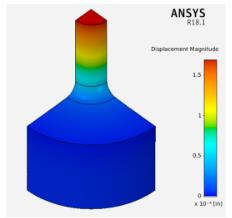## AIM Tutorials

•svecchi
Ansys EmployeeThis tutorial explores the problem of a stepped shaft under an applied axial load which creates an axial stress. Using ANSYS AIM, the axial stress is calculated via simulation and compared to Roark's formula for stress. See the attached file for a tutorial on this problem.

•Vishal Ganore
Ansys Employee

•svecchi
Ansys Employee

The geometry file (CAD) is created in the tutorial by the student. This is done in many of the tutorials, the tutorials which require a geometry will have the appropriate file attached.

•Vishal Ganore
Ansys Employee

Perfect. Thanks.

•tnegem
Subscriber

I am new to Ansys and I am still trying to learn everything I can, Would u please let me know why did you use Hexahedrons instead of just Automatic meshing(which is Trihedrons) ? Also what is the use of deciding of face sizing?

wierd thing is that I am obtaining 1303Psi for Max stress  YY but my equivalent stress is different than your specially the average is twice as high